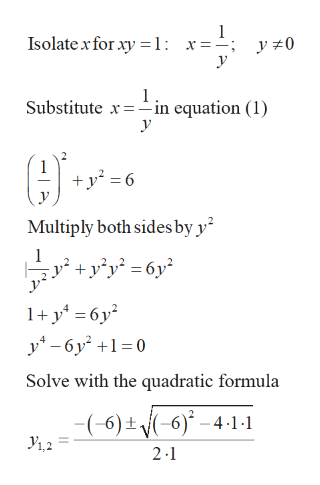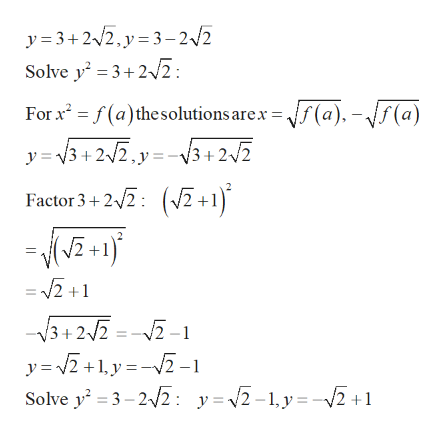# Use any method to solve the nonlinear system. (Order your answers from smallest to largest x, then from smallest to largest y. If there is no solution, enter NO SOLUTION.)x2 + y2 = 6xy = 1(x, y) =         (x, y) =  ‍  (x, y) =     (x, y) =

Question
79 views

Use any method to solve the nonlinear system. (Order your answers from smallest to largest x, then from smallest to largest y. If there is no solution, enter NO SOLUTION.)

 x2 + y2 = 6 xy = 1
(x, y)  =

(x, y)  =

(x, y)  =

(x, y)  =

check_circle

Step 1

Given:

Step 2

Solve the system of equations:help_outlineImage TranscriptioncloseIsolate x for xy = 1: x==; у 1 Substitute x-in equation (1) _ у y2 6 V Multiply both sides by y yy6y 1y6y2 y6 y2 10 Solve with the quadratic formula -(-6)±/(-6)* - 4-1 -1 У,2 2-1 fullscreen
Step 3help_outlineImage Transcriptionclosey 3+22,y 3-2/2 Solve y3+2: For xf(a)thesolutions are.x f(a), -//(a) y 3+ 2/2.yV3+2/2 Factor 3+2: 1) = 2 +1 V3 + 2/Z = --1 Solve y2 3-22: y=2-1,y=-2 +1 fullscreen

### Want to see the full answer?

See Solution

#### Want to see this answer and more?

Solutions are written by subject experts who are available 24/7. Questions are typically answered within 1 hour.*

See Solution
*Response times may vary by subject and question.
Tagged in

### Equations and In-equations